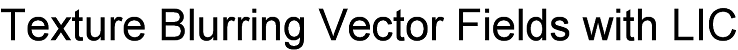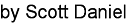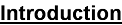Visualizing vector fields has been a well researched problem with applications in fluid dynamics, scientific visualization, and image processing. Three primary approaches to vector field visualization are icon based, particle tracing based, and texture based (Blythe). In this project I focus on using line integral convolutions to blur texture images over a vector field for the purpose of producing novel art effects.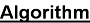The basic algorithm for bluring texture images is conceptually simple. Use a vector field grid and texture image of equal sizes as input and for each pixel calculate a streamline over the texture using data from the vector field. The color values of all the pixels intersected by the streamline are averaged and stored in the originating pixel. Different methods for calculating a streamline are available. A somewhat simple method is to use the Digital Differential Analyzer(DDA) technique to generate straight lines over the texture image as shown in Figure 1. This method, however, doesn't provide the most accurate representation of a vector field.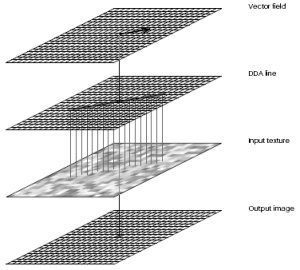The method used to generate streamlines in this project is with line integral convolutions which capture a more detailed representation of vector fields. In this approach each texture pixel is given a unit sized cell and a piecewise line called a line integral of the first kind(LIFK) is used to generate streamlines. The process begins by using the origin pixel's vector data to calculate the length of a streamline from the center of a cell to the edge of a neighboring cell corresponding to a neighboring pixel. Each pixel that is interesected uses its corresponding vector in the vector grid to determine the piecewise direction for that part of the streamline. This continues for each intersected pixel until a certain streamline length is attained. The color values of intersected pixels is averaged and stored as before.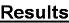I broke down my project into two parts. The first few images show the interface of my vector field creation program. The image that is going to be blurred is placed in the background for convenience. A second program does the actual line integral convolutions and provides a mode for visualizing vector data. The remaining images show the results of my line integral convolutions over various textures.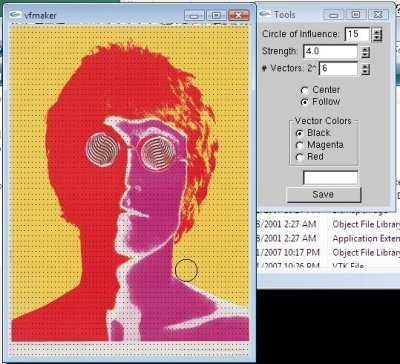Figure a. Initial vector creation interface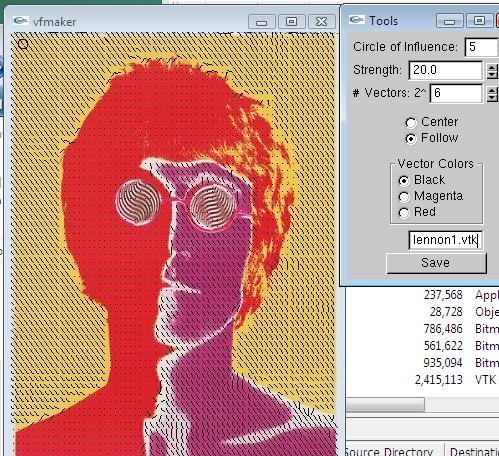Figure b. Image with manipulated vectorsFigure c. Vector visualization from dataset in left image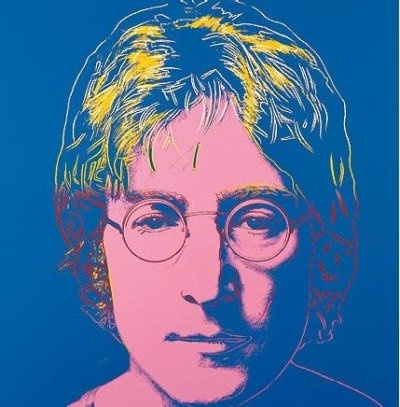Figure d. Original image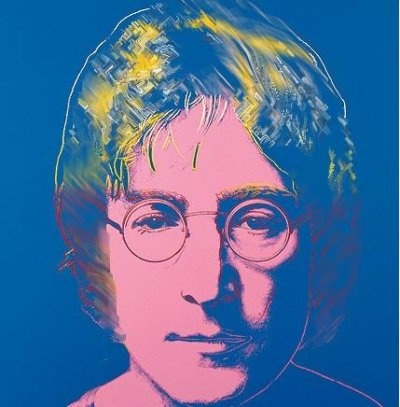Figure e. Image with LIC applied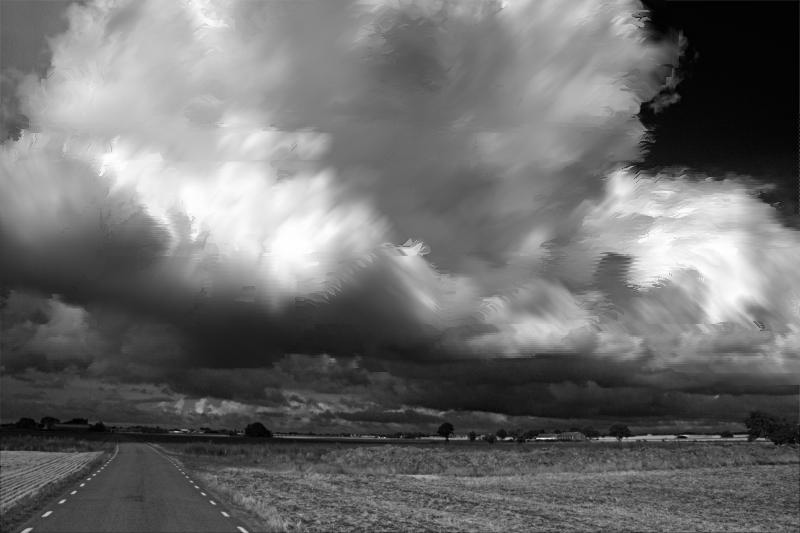Figure g. Cloud blur to soften contrast between light and dark spots.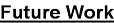The are several additions and enhancements that can be made to my project.
1. Line inegral convolution is computationally expensive and can take a long time to complete for even 300x300 sized textures. A faster method is proposed by Stalling in the paper listed below.
2. Higher order curves can be also be used as streamlines. Rather than use a piecewise line of straight segments cubic or Bezier lines might be better suited for capturing curvature in vortices.
3. Cabral and Leedom note another method for generating vector data by using the input image itself to define a vector field. Such an approach would be an interesting way to explore directional information inherent in an image.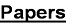Cabral, Brian, Leith Leedom. "Imaging Vector Fields Using Line Integral Convolution." March, 2007. <paper>

Stalling, Detlev, Hans-Christian Hege. "Fast and Resolution Independent Line Integral Convolution". March, 2007 <paper>